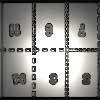#### You may also like### Exploring Wild & Wonderful Number Patterns

EWWNP means Exploring Wild and Wonderful Number Patterns Created by Yourself! Investigate what happens if we create number patterns using some simple rules.### Magazines

Let's suppose that you are going to have a magazine which has 16 pages of A5 size. Can you find some different ways to make these pages? Investigate the pattern for each if you number the pages.### Pebbles

Place four pebbles on the sand in the form of a square. Keep adding as few pebbles as necessary to double the area. How many extra pebbles are added each time?

# The Mathemagician's Seven Spells

##### Age 7 to 11 Challenge Level:

We have had an overwhelming reponse to this problem. Thank you to everyone who sent in a solution.

Particularly well-explained answers came from Tilly at Maldon Court Preparatory School, Alistair from Histon and Impington Infant School, Caroline from Tattingstone School, Samuel from Bispham Drive Junior School, Miss White's Class, Lucy from The King's Primary School, students from Penrice Community College, Chris and Keisha from Alton Park, Sean, Jack and Joe from Devonshire Primary, James and Josh from Thornton Dale Primary, Luke and Blake from Park House School, pupils from St Teresa's Catholic Primary School, children from Brewood Middle School and Saloni who didn't give a school.

Let's look at the spells in turn:

1. $1, 2, 4, 8, 16, 32.$
"We are doubling the number before it," Alistair explained.

2. $6, 13, 20, 27, 34, 41$
Tilly wrote here that we are adding $7$ to the previous number.
Samuel adds, "I worked out my $7$ times table to help me because all the numbers are one less than multiples in the $7$'s table."

3. $127, 63, 31, 15, 7, 3$
There were several different ways to work this one out:
"We are halving and taking away $0.5$ from the answer," said Alistair. Lucy also tackled this one in the same way.
"The differences were halving themselves this time. The difference between $127$ and $63$ is $64$; the difference between $63$ and $31$ is $32$; the difference between $31$ and $15$ is $16$ so I knew that the next differences would be $8$ then $4$," explained Samuel. Saloni also tackled it this way.
Miss White's class suggested that you take away $1$ then divide by $2$. David put this slightly differently by saying, "$-1\times 0.5.$"

4. $1, 3, 6, 10, 15, 21, 28$
Samuel suggests: "This time the difference increased by one each time. The difference between $1$ and $3$ is $2$; between $3$ and $6$ is $3$; between $6$ and $10$ is $4$; between $10$ and $15$ is $5$ so I knew that the next differences would be $6$ and $7$."
Miss White's class put it slightly differently, "Between the numbers there is a pattern of addition. You first add $2$, then $3$, then $4$ and so on.
Saloni spots that these are all triangular numbers.

5. $2, 3, 6, 11, 18, 27, 38$
David points out the pattern is $+1 +3 +5 +7 +9 +11 +13$ etc. Miss White's class pointed out that the numbers added are the odd numbers in order.

6. $1, 8, 27, 64, 125, 216$
Alistair says, "I spotted that several of the numbers were multiples, so I started by looking for factors before I spotted the answer. The $nth$ term in the series would be $n\times n\times n$ (or n cubed)."
Samuel looked at the difference between the numbers: This was the hardest one to solve. The numbers increased by $7, 19, 37$ but I could not see a pattern so I looked at a pattern with $7, 19, 37$. I saw that the differences between these numbers were $12$, and $18$, and were increasing by $6$ each time. So I added $6$ onto $18$ which gave $24$ then added $24$ on to $37$ which gives $61$. Next I took $61$ and added it to the $64$ which gave $125$. With the $24$ I again added $6$ which gave $30$ so I added $30$ to $61$ which gave $91$. I added $91$ to $125$ which gave a final number of $216$."
Caroline, like Alistair, realised that all the numbers were cubes. "I just did $5\times 5\times 5$ and $6\times 6\times 6$ to get the answers for the next two numbers," she said. Lucy also knew these were cubic numbers.
Chris and Keisha from Alton Park suggested that you "add on every fourth prime number".

7. $216, 168, 126, 90, 60, 36, 18, 6$
As many of you found, this was hard to explain. Samuel has done an excellent job:
What I did for the final spell was I found the difference between $216$ and $168$ which was $48$, between $168$ and $126$ which was $42$, between $126$ and $90$ which was $36$. I noticed that these differences decreased by $6$ so I took $6$ from $24$ ($60 - 36$) which gave me $18$. Then I took $18$ from $36$ which is $18$ and so this is the next number in the sequence. Similarly, I took $6$ from $18$ (the last difference) which gave me $12$ and then I took $12$ from $18$ (the $18$ in the sequence) so the final answer is $6$.
Alistair had a slightly different method: I found the factors of the numbers, and spotted that all are divisible by $6$. When I had divided by $6$, the series was $36, 28, 21, 15, 10, 6$ and the differences went down by one each time.

Very well done!